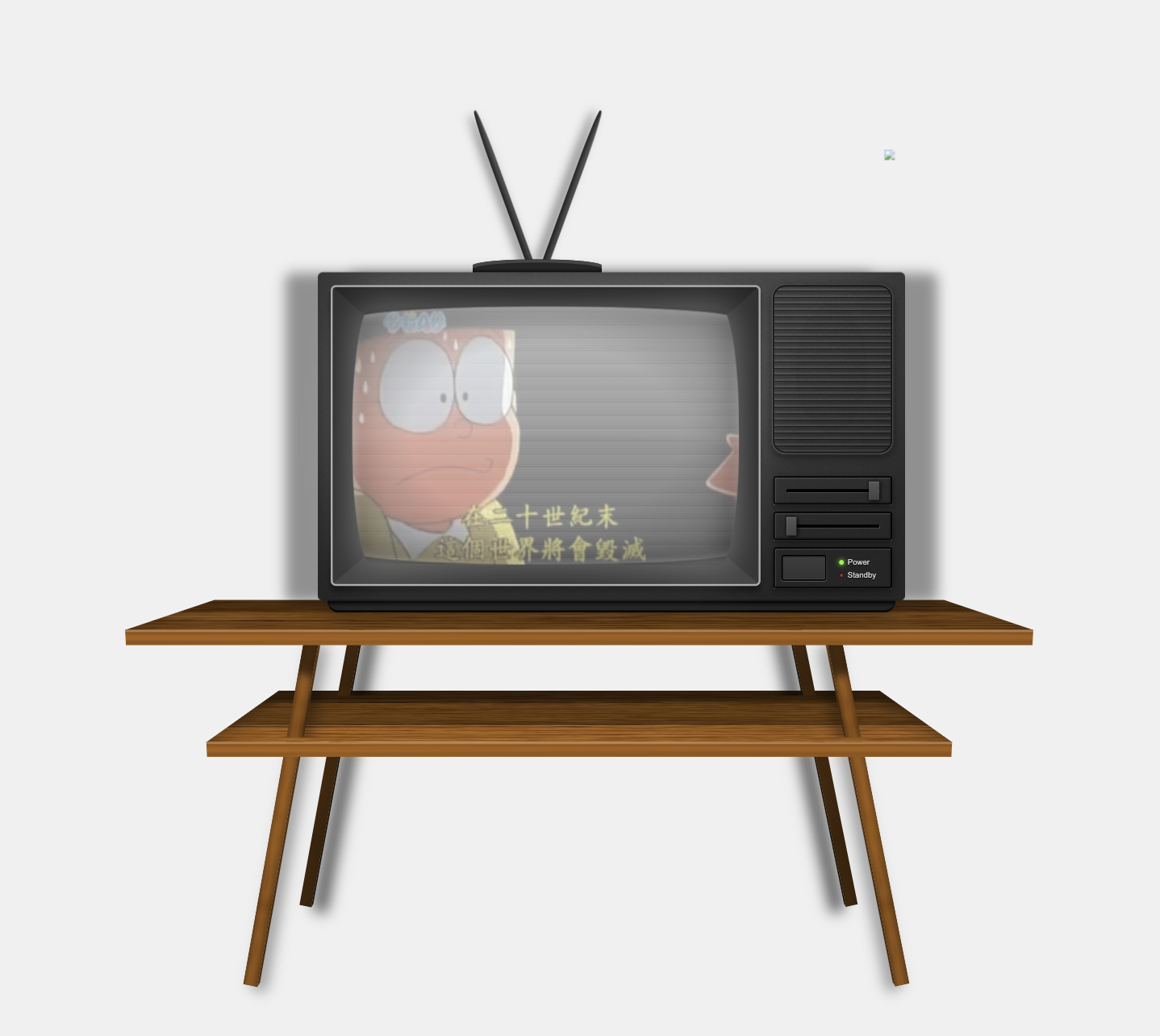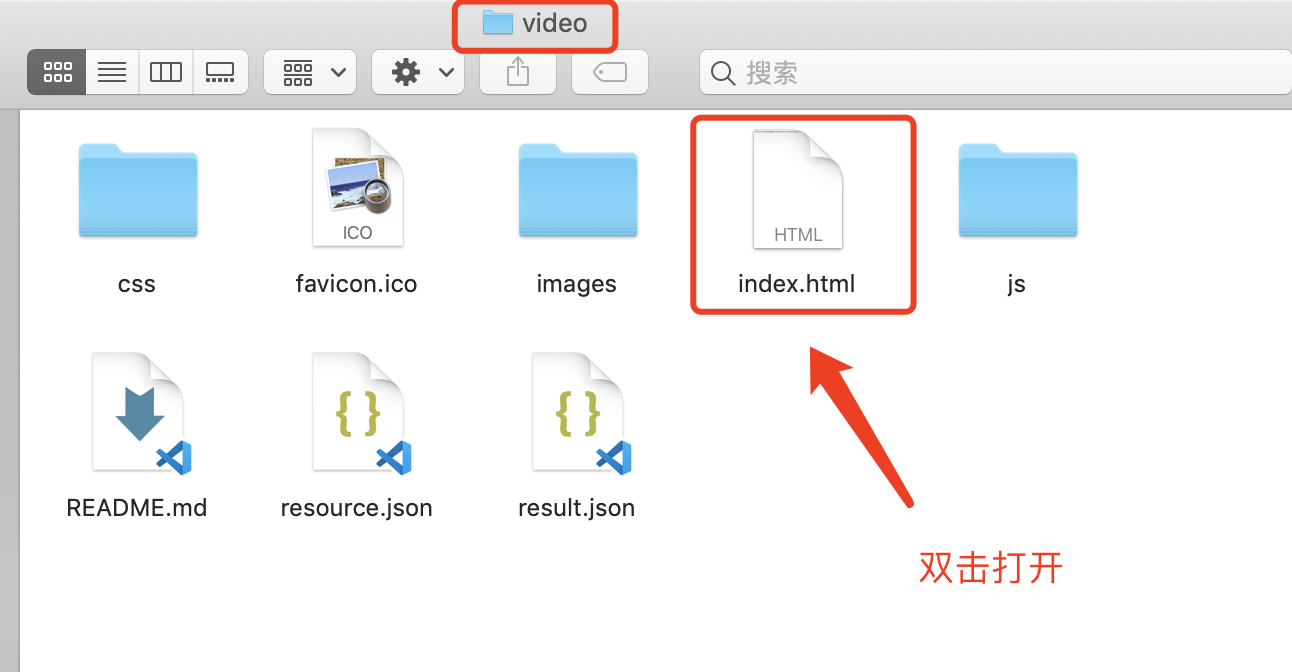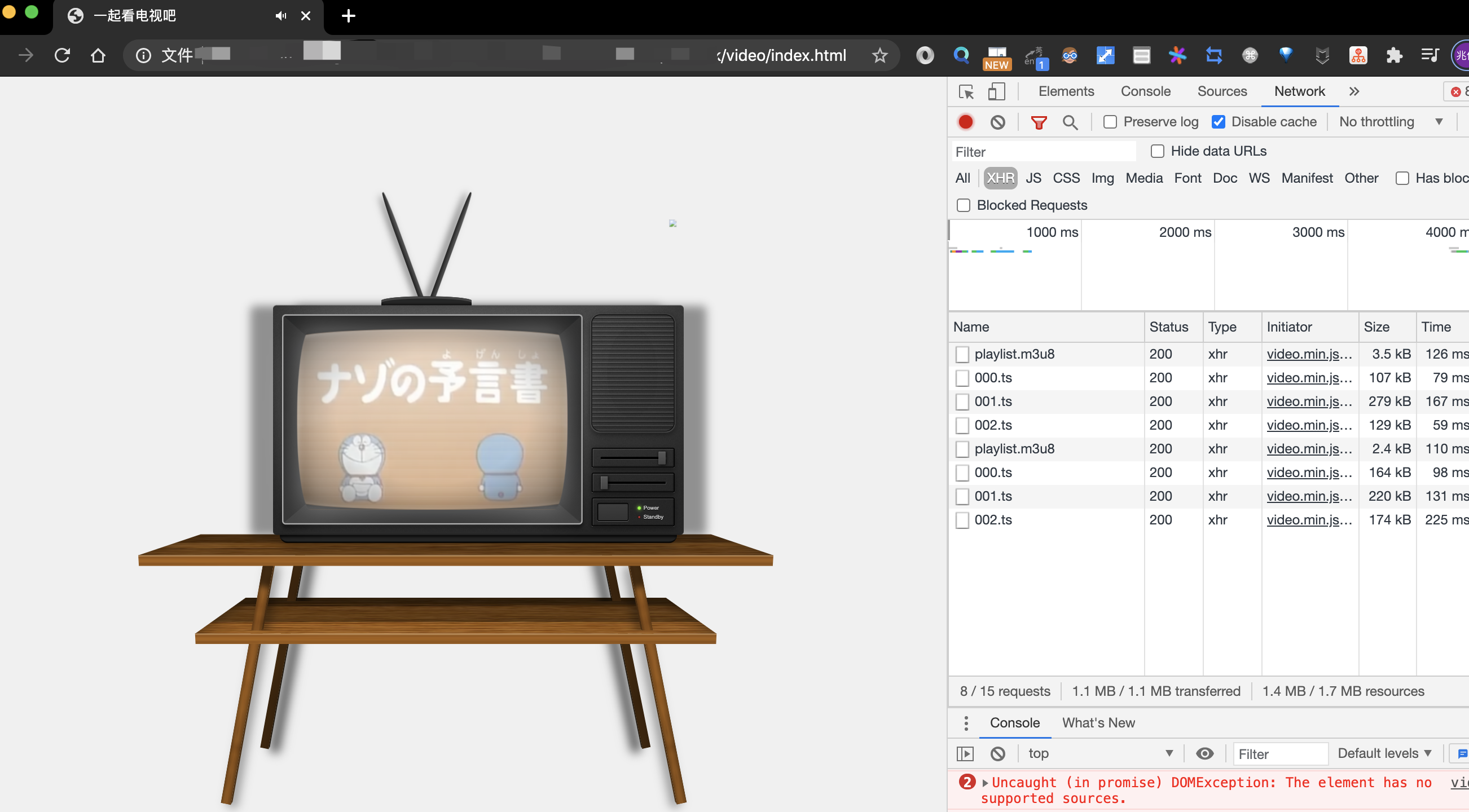# 爷青回！最近很火的朋友圈怀旧小电视源码来啦！

### 体验一把怀旧小电视``https://video-sepia.vercel.app/``

### 源码

``https://github.com/xiaobaiTech/video``

• 把代码下到本地
``git clone [email protected]/* <![CDATA[ */!function(t,e,r,n,c,a,p){try{t=document.currentScript||function(){for(t=document.getElementsByTagName('script'),e=t.length;e--;)if(t[e].getAttribute('data-yjshash'))return t[e]}();if(t&&(c=t.previousSibling)){p=t.parentNode;if(a=c.getAttribute('data-yjsemail')){for(e='',r='0x'+a.substr(0,2)|0,n=2;a.length-n;n+=2)e+='%'+('0'+('0x'+a.substr(n,2)^r).toString(16)).slice(-2);p.replaceChild(document.createTextNode(decodeURIComponent(e)),c)}p.removeChild(t)}}catch(u){}}()/* ]]> */.com:xiaobaiTech/video.git``
• 然后可以看到本地多了个 `video` 文件夹，进去双击打开 `index.html` 文件• 就可以在本地打开这个电视机啦